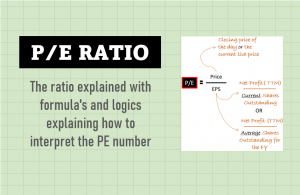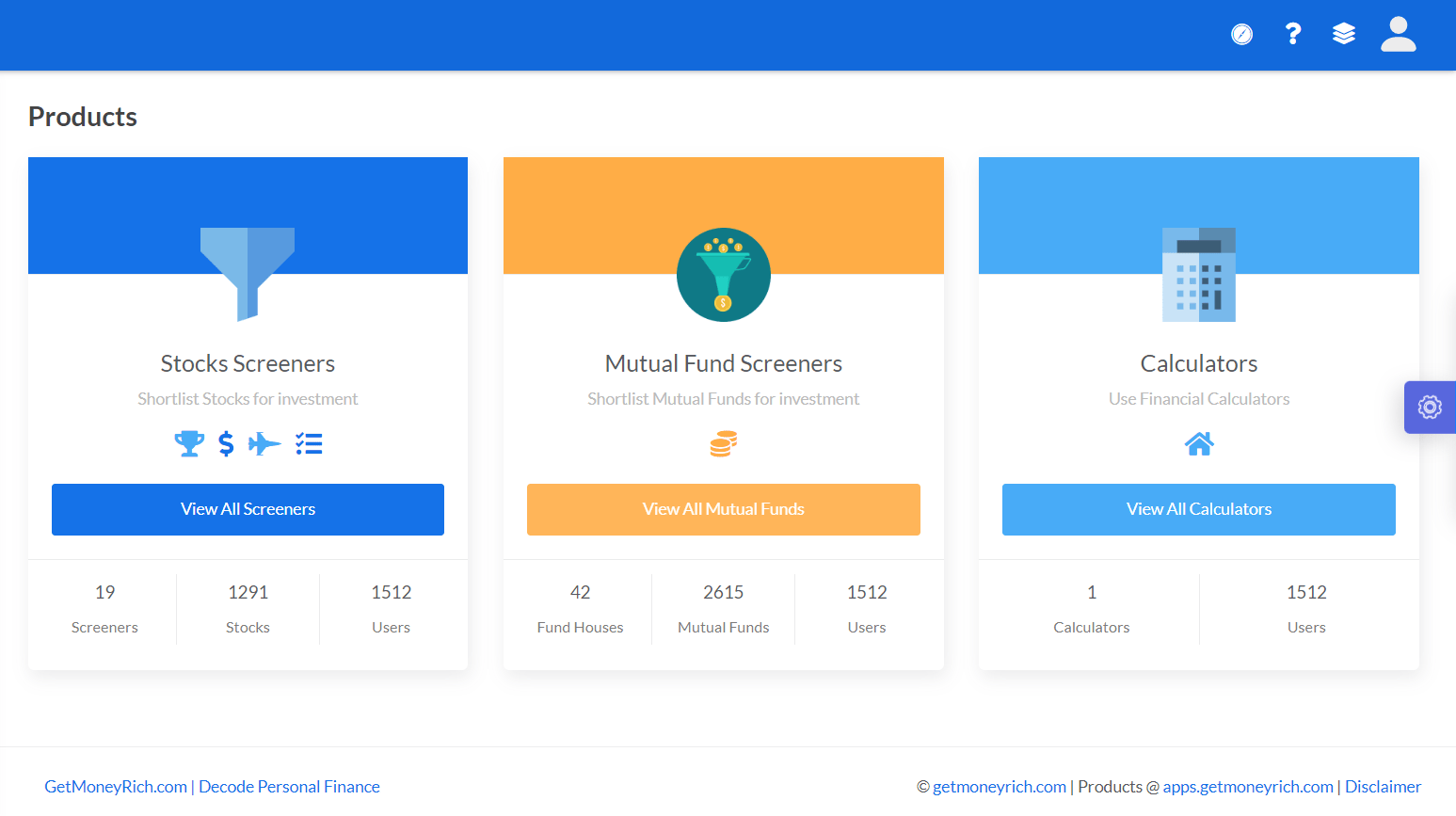# EV/EBITDA Multiple – How To Calculate Target Price Using It

In this article, we will talk about the EV/EBITDA multiple. It is also known as Enterprise Value (EV) multiple. To get a better understanding of the Enterprise Value, I’ll suggest you kindly read this article.

EV/EBITDA ratio is very similar to the P/E Ratio. Though PE ratio is one of the most widely used financial Ratios, the use of EV/EBITDA is more effective. EV/EBITDA multiple is a more apt representation of the company’s valuation.

Use of P/E ratio and EV/EBITDA multiple at tandem is advisable. As an investor, to judge a company’s acquisition valuation, the use of the EV/EBITDA ratio is better. It gives a more realistic feel about the fair value of the company.

Before we get into the detail of how to calculate and interpret the EV/EBITDA multiple, let’s get some basics clear. First, I would like to explain the relationship between a P/E and EV/EBITDA ratio.

## Similarities Between P/E and EV/EBITDA Ratio

P/E ratio and EV/EBITDA may sound like two different ratios, but they have a common root. As you can see in their formulas, the EV/EBITDA ratio is like an extension of the P/E Formula.

P/E = Market Cap / Net Profit.

EV/EBITDA = (Market Cap + Total Debt – Cash & Cash Equivalent) / (Net Profit + Interest + Tax + Depreciation)

Both ratios have two components, ‘company’s value’ in the numerator and ‘profit’ in the denominator. But the difference lies in what is considered as a company value and profit to calculate the ratio.

The below infographic will highlight the components of the P/E Ratio first, and then we will do a similar analysis for EV to EBITDA ratio.

#### PE Ratio

P/E ratio mainly estimates the company’s value based on its equity component (shares x market price). While the profit number is estimated by accounting for all operational and non-operation expenses (net profit).

This is one way of expressing the company’s valuation. The other way of doing it is by using the EV and EBITDA values.

#### EV/EBITDA Ratio

• Enterprise Value (EV): It estimates the total acquisition value of a company after considering its net-debt load. What is net debt? It is total debt minus cash & cash equivalent. Why consider debt? Because upon acquisition, the company’s debt (& Cash) also gets transferred and will become a cost/liability for the purchaser.
• EBITDA: The benefit of using EBITDA in the denominator is that it accounts for only operational costs. It is a better representation of the profitability of a business than Net Profit. Why? Because it does not count non-operational costs like interest, tax, and depreciation.

## How to Calculate EV to EBITDA Ratio?

Let’s try to calculate the EV to EBITDA ratio of our example company ABC. There will be three stages of the calculation:

1. Market Cap: To calculate market capitalization, first note down share price (P=1070) and nos of shares outstanding (N=633.93 Cr.). Then multiplying P x N will give the market capitalization. For our example company, the market cap will be Rs.6,78,305 Crore (=1070 x 633.93).
2. EBITDA: Note net profit (PAT), and adding to it the following non-operational expenses: tax, interest, and depreciation will give EBITDA. Our example company has an EBITDA of Rs.62,149 Cr.
3. Enterprise Value (EV): It is calculated by noting the market capitalization and then adding net debt (debt – cash). For our example company, the market cap is Rs.6,78,305, and net debt is Rs.2,21,584 Cr. (=2,30,027-8,443). Hence EV will be 8,99,889 Cr. (=6,78,305+2,21,584).

We are now ready will all the values required to calculate the ratio. Just use the EV and EBITDA numbers to do the calculation. As I’ve shown in the above calculations, the ratio for our example company is 14.48 (=8,99,889/62,149)

## Which is a better ratio P/E, or EV/EBITDA?

P/E ratio is a more widely used ratio among the two. But this is because it is easier to calculate the P/E Ratio than the EV/EBITDA multiple. Moreover, the P/E Ratio is also readily available for stocks in all major financial dailies and online journals. But the later ratio is not as readily available.

Having said that, I would also like to reiterate that EV/EBITDA is a much more effective multiple for stock’s value judgment. Let me explain it using a hypothetical example.

Suppose there are two hypothetical companies ABC and DEF. Shares of both these companies are trading at a market price of Rs.250, and their Earning Per Share (EPS) is Rs.20. Hence, in this case, the P/E ratio for both ABC and DEF will be 12.5 (=250/20). As both the companies have the same P/E and EPS, we might consider both as evenly priced, right?

But this might be a misguided assumption. Why? Because of the ‘EPS’ factor. How to calculate EPS? Net Profit/shares. Many non-operational factors might change the net profit number. Three such factors that immediately come to mind are depreciation, share buyback, and deferred tax policy.

##### #1. Effect of Depreciation on Net Profit

Let’s take the company ABC for example. The same company can have two different net profit numbers for the same FY. This can happen if the management decides to take an alternative policy decision on depreciation deduction. Let me show you how…

In case ABC (1), the company has considered a Depreciation deduction of only 8,000. But in the second case ABC (2), the depreciation deduction of 18,000 was considered. This can happen if the company opts for straight-line depreciation benefits vs an accelerated depreciation benefit respectively.

So what is the result? The result is, in the case of ABC (1) Net profit was 57,400. In the case of ABC (2), the net profit number slumped to 50,400. This is a fall of more than 12%.

The point is that without any contributions from the operations, the company would post less or high net profit numbers based on their policy. That is like ambiguity or unpredictable area for investors. Hence, professional investors do not like to rely too much on PAT numbers posted by the companies. They have more faith in EBITDA (operating profit) numbers.

##### #2. Effect of Share Buyback on EPS

Lowering of P/E is an indication of improving earning or falling-price. In both the case, it indicates a favorable change of fundamentals. But there is a way to fool the investors by lowering P/E by forced share buyback. That reduces the shares outstanding, thereby EPS goes up, and P/E falls.

It is a way to artificially lower the P/E without any real change in the fundamentals. But the EV/EBITDA ratio cannot be influenced by outside activities. Hence, EV to EBITDA ratio is more reliable.

##### #3. Effect of Deferred Tax on Net Profit

It is also possible to influence the net profit of the company by a deferred tax policy. A company may decide to show more net profits than actual by choosing to defer their tax liability in the future. Similarly, net profits can also be lowered by intentionally paying more tax than necessary.

So you can see, without any change in profits at the operational level, a policy decision by the management (agreeing to deferred tax liability) can enhance the net profit.

But if we are dealing in EBITDA instead of net profit (PAT), such artificial influence is not possible.

## Quality of EV to EBITDA Ratio

As an investor, we should not rely only on a one year EV/EBITDA number. We must track the history of this ratio. What we would like to see is a reducing ratio. This is step number one.

The next step is to further deeply check what has caused the lowering of the ratio.

We would like to see a reducing ratio due to an increase in EBITDA or a reduction in net debt, or both.

If the ratio is falling due to market cap reduction, then it will need further checks. If the reason is a fall in price, then check the growth rates. The parameter to be checked can be sales, net profit, EPS, net worth, cash flow, etc.

## Conclusion

Low P/E ratio and low EV/EBITDA multiple are both good indicators of undervaluation. But considering the comparative ease with which net profits and EPS of a company can be influenced, EV/EBITDA looks more reliable.

Under normal circumstances, both P/E and EV/EBITDA numbers are reliable. But if you doubt the quality of the company’s earnings, then go for the EV/EBITDA ratio. This ratio also has its limitations, but it is certainly more transparent than the P/E Ratio.

My stock analysis worksheet provides a historical P/E and EV to EBITDA ratio for the last 10 years of a company. This helps me to see the price valuation of the company by being more skeptical 🙂#### MANI

Hi. I’m Mani, I’m an Engineering graduate who in pursuit of financial independence, has converted into a full time blogger. After working in the corporate world for almost 16+ years, I bid it adieu....read more### Price To Earnings Ratio [P/E] – Basics, Formula, Calculation, & Interpretation

P/E ratio in its basic form (trailing P/E) may not be as useful. But there can be multiple derivaties of it. P/E ratio seen through the lens of ‘earning yield’ or ‘PEG ratio’ gives good insights into price valuation. I personally love valuing high P/E stocks using the ‘forward P/E’ method.

### 3 Responses

1.Gopi Kuppanna says:

Hi Mani,
Thanks for all the gyan shared in your blog. Great knowledge sharing. Kudhos.
In the above article I did not get how to calculate target price from the ratio. From the title, I assume you will summarize that part. What is the best way to calculate target price for a stock if I decide to invest in it?

2.satish says:

Dear Mani,
Your articles are excellent and I am happy for you that you are following your passion. I have been following the stock market for quite some time but starting to understand more now. I have a Product Idea and would like to discuss with you. As far as I know, this kind of product is not available in the market. Please ping me on the email if you are interested to discuss.

Thanks

1.MANI[sh] says: